1. /
2. CBSE
3. /
4. Class 08
5. /
6. Mathematics
7. /
8. NCERT Solutions for Class...

# NCERT Solutions for Class 8 Maths Exercise 11.1### myCBSEguide App

Download the app to get CBSE Sample Papers 2023-24, NCERT Solutions (Revised), Most Important Questions, Previous Year Question Bank, Mock Tests, and Detailed Notes.

NCERT solutions for Class 8 Maths Mensuration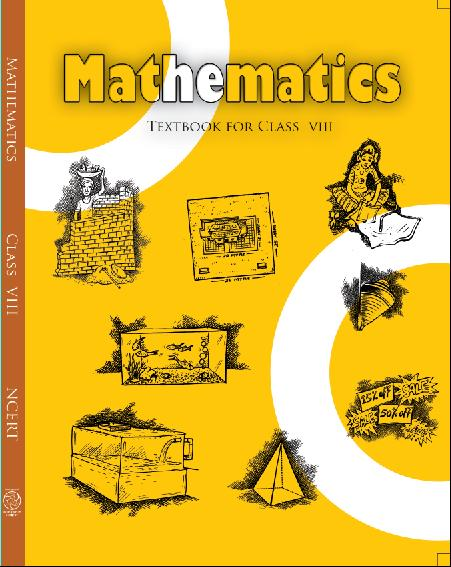## NCERT Solutions for Class 8 Maths Mensuration

###### 1. A square and a rectangular field with measurements as given in the figure have the same perimeter.

Which field has a larger area?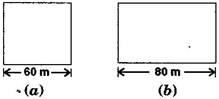Ans. Given: The side of a square = 60 m

And the length of rectangular field = 80 m

According to question,

Perimeter of rectangular field

= Perimeter of square field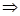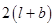=  4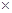side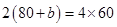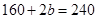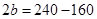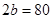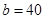m

Now Area of Square field

=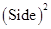=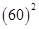= 3600 m2

And Area of Rectangular field

= lengthbreadth = 8040

= 3200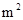Hence, area of square field is larger.

NCERT Solutions for Class 8 Maths Exercise 11.1

###### 2. Mrs. Kaushik has a square plot with the measurement as shown in the figure. She wants to construct a house in the middle of the plot. A garden is developed around the house. Find the total cost of developing a garden around the house at the rate of  55

per m2.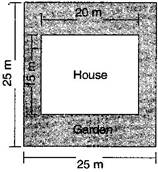Ans.  Side of a square plot = 25 m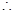Area of square plot ==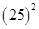= 625 m2

Length of the house = 20 m and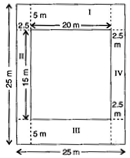Breadth of the house = 15 mArea of the house = lengthbreadth

= 2015 = 300 m2

Area of garden = Area of square plot

– Area of house

= 625 – 300 = 325 m2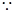Cost of developing the garden per sq. m =  55Cost of developing the garden 325 sq. m =  55325

=  17,875

Hence total cost of developing a garden around is ` 17,875.

NCERT Solutions for Class 8 Maths Exercise 11.1

###### 3. The shape of a garden is rectangular in the middle and semi-circular at the ends as shown in the diagram. Find the area and the perimeter of this garden [Length of rectangle is 20 – (3.5 + 3.5 meters]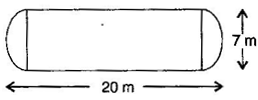Ans. Given: Total length = 20 m

Diameter of semi circle = 7 mRadius of semi circle =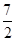= 3.5 m

Length of rectangular field

= 20 – (3.5 + 3.5) = 20 – 7 = 13 m

Breadth of the rectangular field = 7 mArea of rectangular field =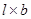= 137 = 91Area of two semi circles =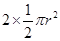=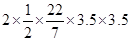= 38.5 m2

Area of garden = 91 + 38.5 = 129.5 m2

Now Perimeter of two semi circles =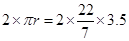= 22 m

And Perimeter of garden

= 22 + 13 + 13

= 48 m

NCERT Solutions for Class 8 Maths Exercise 11.1

###### 4. A flooring tile has the shape of a parallelogram whose base is 24 cm and the corresponding height is 10 cm. How many such tiles are required to cover a floor of area 1080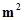? [If required you can split the tiles in whatever way you want to fill up the corners]

Ans. Given: Base of flooring tile = 24 cm

= 0.24 m

Corresponding height of a flooring tile

= 10 cm = 0.10 m

Now Area of flooring tile

= BaseAltitude

= 0.240.10

= 0.024 m2Number of tiles required to cover the floor

=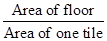=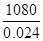= 45000 tiles

Hence 45000 tiles are required to cover the floor.

NCERT Solutions for Class 8 Maths Exercise 11.1

###### 5. An ant is moving around a few food pieces of different shapes scattered on the floor. For which food-piece would the ant have to take a longer round? Remember, circumference of a circle can be obtained by using the expression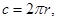where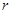is the radius of the circle.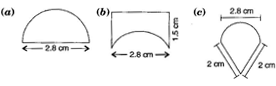Ans. (a) Radius =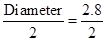= 1.4 cm

Circumference of semi circle =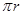=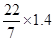= 4.4 cm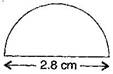Total distance covered by the ant

= Circumference of semi circle + Diameter

= 4.4 + 2.8 = 7.2 cm

(b) Diameter of semi circle = 2.8 cm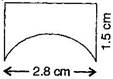Radius =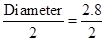= 1.4 cm

Circumference of semi circle === 4.4 cm

Total distance covered by the ant

= 1.5 + 2.8 + 1.5 + 4.4 = 10.2 cm

(c) Diameter of semi circle = 2.8 cm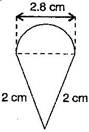Radius == 1.4 cm

Circumference of semi circle === 4.4 cm

Total distance covered by the ant

= 2 + 2 + 4.4 = 8.4 cm

Hence for figure (b) food piece, the ant would take a longer round.

## NCERT Solutions for Class 8 Maths Exercise 11.1

NCERT Solutions Class 8 Mathematics PDF (Download) Free from myCBSEguide app and myCBSEguide website. Ncert solution class 8 Mathematics includes text book solutions from Class 8 Maths Book . NCERT Solutions for CBSE Class 8 Maths have total 16 chapters. 8 Maths NCERT Solutions in PDF for free Download on our website. Ncert class 8 solutions PDF and Maths ncert class 8 PDF solutions with latest modifications and as per the latest CBSE syllabus are only available in myCBSEguide.

## CBSE app for Class 8

To download NCERT Solutions for class 8 Social Science, Computer Science, Home Science,Hindi ,English, Maths Science do check myCBSEguide app or website. myCBSEguide provides sample papers with solution, test papers for chapter-wise practice, NCERT solutions, NCERT Exemplar solutions, quick revision notes for ready reference, CBSE guess papers and CBSE important question papers. Sample Paper all are made available through the best app for CBSE students and myCBSEguide website.Test Generator

Create question paper PDF and online tests with your own name & logo in minutes.myCBSEguide

Question Bank, Mock Tests, Exam Papers, NCERT Solutions, Sample Papers, Notes

### 13 thoughts on “NCERT Solutions for Class 8 Maths Exercise 11.1”

1. It’s amazing

2. Good
Nice good

3. thnx it helped me alot in my studies actually i was hesitated bcz of que no 3 and by trick i found the ans but it helped me check my process and the mostake i have done it also helped me in completing my work

4. Thanks for this great help. I am very much thankful and grateful to u for this help.

5. Very good and very well

6. Thanx for this help

7. Perfectly
anyone can understand easily

8. very helpful and open easly this is a best wabsite for reading
thanks

9. Thanks